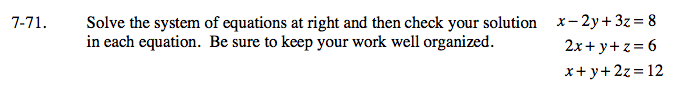Home > A2C > Chapter 7 > Lesson 7.1.5 > Problem7-71

7-71.
1. Solve the system of equations below and then check your solution in each equation. Be sure to keep your work well organized. Homework Help ✎ x − 2y + 3z = 8
2x + y + z = 6
x + y + 2z = 12Choose a variable to eliminate and two equations to work with.
There are many possibilities.
We will use the first and third equations and eliminate x in the example.
Multiply the third equation by −1 then add it to the first.

Choose a different pair of equations and eliminate the same variable.
Here, we use the second and third equations.
Multiply the third equation by −2 then add it to the second.

Now combine the two result equations.

x − 2y + 3z = 8
xy − 2z = −12
−3y + z = −4

2x + y + z = 6
−2x − 2y − 4z = −24
y − 3z = −18

−3y + z = −4
3y + 9z = 54

Solve for z and substitute to find the other values.

Check your result in each equation.